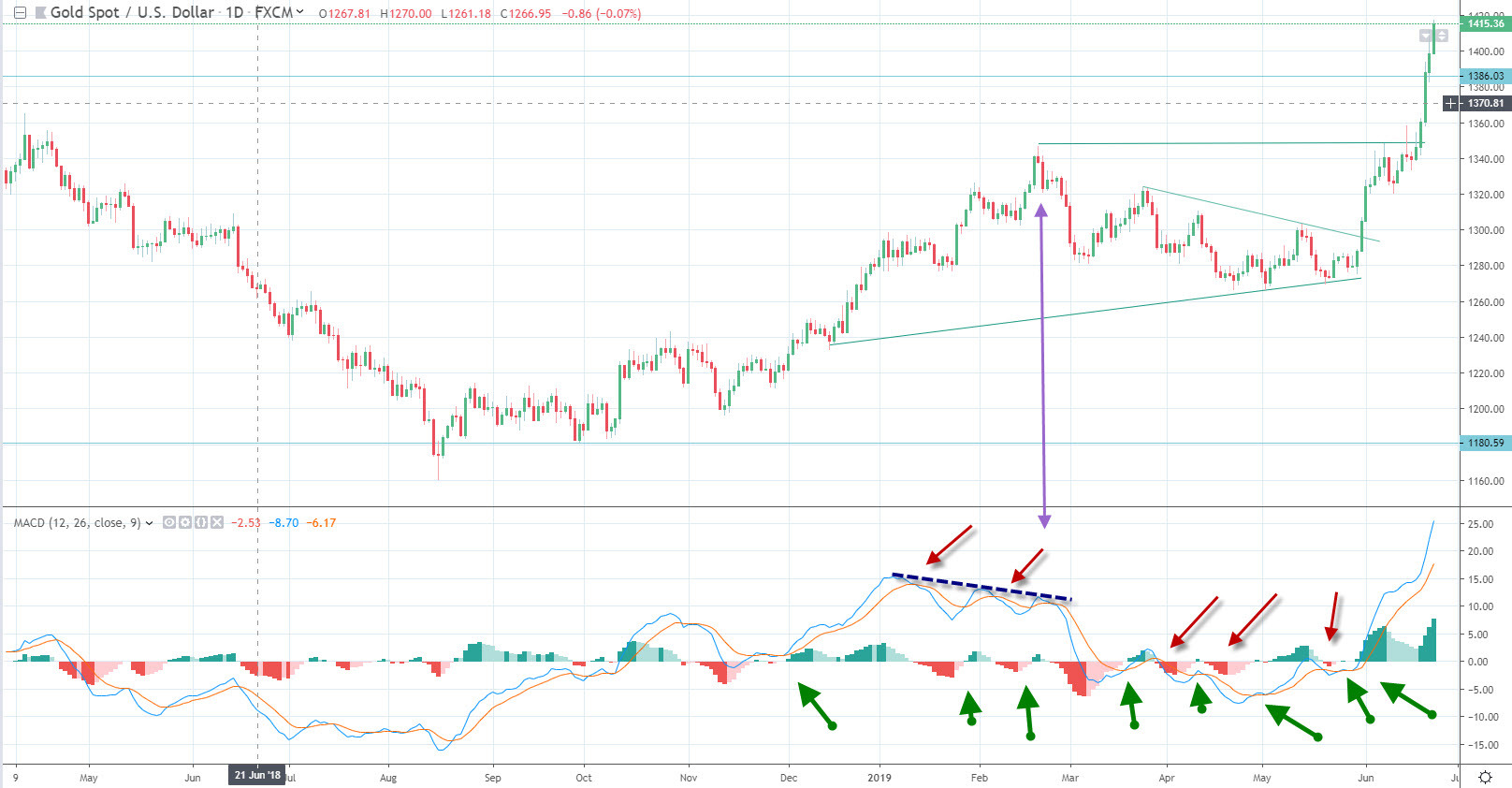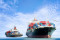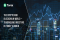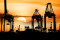FXEMPIRE
All

# Technical Analysis MACD – How Professional Traders Use it

Momentum is one of the most important concepts use to generate strategies by professional traders. As momentum accelerates the price of an asset can break out or break down, signally to traders that a trend is beginning.When momentum begins to fall it signal to traders that prices could begin to consolidate or reverse. One of the best and most complete momentum indicators is the MACD (moving average convergence divergence) index. Many traders also use the MACD histogram to forecast MACD crossover signals.

## History of the MACD

The MACD was created by Gerald Appel in the late 1970’s. The MACD measures momentum by measuring whether certain moving averages are converging or diverging. The MACD is considered one of the simplest and most effective momentum indicators available. The MACD calculates momentum by subtracting the longer moving average from a shorter moving average.

## The Components

The nuts and bolts of the MACD consist of a 12-day exponential moving average, and the 26-day exponential moving average. The MACD line, is created by subtracting the 12-day exponential moving average from the 26-day exponential moving average. The MACD signal line is the 9-day exponential moving average of the MACD line. The MACD histogram is the difference between the MACD line and the MACD signal line.

## The Crossover Signal Strategy

Divergence occurs when the 2-exponential moving average moves away from one another. Convergence occurs when the moving averages move towards each other. The shorter moving average (the 12-day exponential moving average is faster and responsible for most MACD movements. The longer moving average (the 26-day exponential moving average) is slower and less reactive to price changes.

When the MACD line crosses above the MACD signal line positive momentum is accelerating. When the MACD line crosses below the MACD signal line negative momentum is accelerating. You can use the crossover signal as a straight buy signal, but you can also incorporate this signal with other technical analysis tools.You can see from the chart of gold prices that there are several MACD signals that are generated and when the price of gold is moving sideways the signals made it difficult to generate gains. On the other hand, when a trend breaks out, the MACD quickly exposes accelerating momentum, as does not turn back.

## MACD Histogram

The MACD signal line can also be viewed in histogram form. The MACD histogram oscillates around the zero-index level. When the histogram crosses above the zero-index line a buy, a signal is generated. When the MACD histogram crosses below the MACD zero index level a sell signal is generated. The MACD-Histogram represents the difference between MACD and its 9-day EMA, the signal line. The histogram is positive when MACD is above its 9-day EMA and negative when MACD is below its 9-day EMA.

MACD divergence occurs when prices are rising or falling at a decelerating rate. This concept is important as you want to ride the trend of the market when price changes are accelerating. When prices begin to decelerate the market is moving into equilibrium and will likely consolidate until new information moves it again.

The way to measure a MACD divergence is to look for a situation where prices are rising and the MACD trajectory is falling. It could also occur when prices are falling and the MACD trajectory is rising.

## Examples of Divergence

If you look at the chart of gold prices you can see that a MACD divergence occurred in February of 2019. Here the prices of gold were rising but the trajectory of the MACD line was declining. You can draw a trend line of the MACD line to determine the trend of the MACD line. You can also perform this analysis using the MACD histogram. If the price of gold is rising but the MACD histogram is flat or declining than a divergence is occurring.

Prices in these situations are rising while momentum is falling, which means that prices are experiencing a blow off. A good way to think about this is when you take your foot off the gas of a car when you are climbing up a hill. At first, you would continue higher, but as your rate decelerates, you eventually get to a point where you might begin to slide backward.

## Using the MACD histogram to Forecast a Crossover

The MACD-Histogram can help you forecast a signal line crossover in MACD, when divergences are started to occur. These divergences signal that MACD is converging on its signal line and could be poised to generate a crossover signal. There are two types of divergences, peak-trough and slant. A peak-trough divergence forms with two peaks or two troughs in the MACD-Histogram. A peak-trough bullish divergence forms when MACD forges a lower low and the MACD-Histogram forges a higher low. Well-defined troughs are important. Slant divergence i like the divergences you see when you draw a trend line through the MACD line.

## Summary

The Moving Average Convergence Divergence index is one of the best and most efficient momentum oscillators. The index is formed by deriving the difference between two moving average to determine if prices of an asset are accelerating or decelerating. The most common signal used by traders is the crossover buy and sell signal. In addition, many traders use the MACD histogram. This is the difference between the MACD line and the MACD signal line. When prices crossover the zero-index level a signal is generated. Traders also use divergence. This is when prices are moving one way and the MACD fails to confirm acceleration. There are two different types of divergence, the first is peak through and the second is slant.

Forex4you

Don't miss a thing!

## Latest Articles

See All

See All### Popular

SymbolPRICEChange
EURUSDEUR/USD
GBPUSDGBP/USD
XAUUSDGold
CLCrude Oil
SPXS&P 500